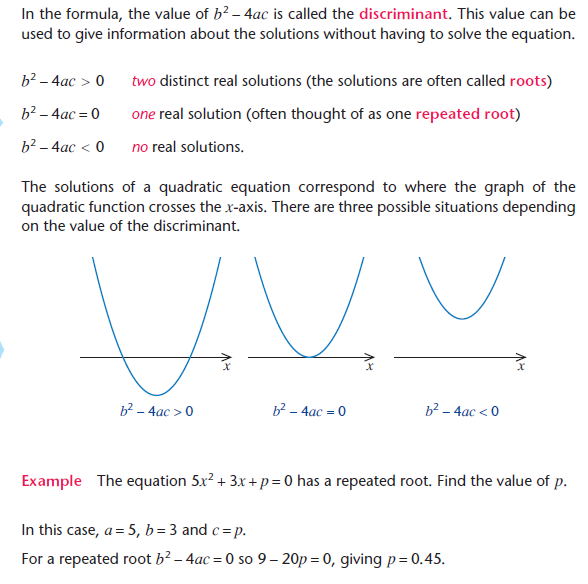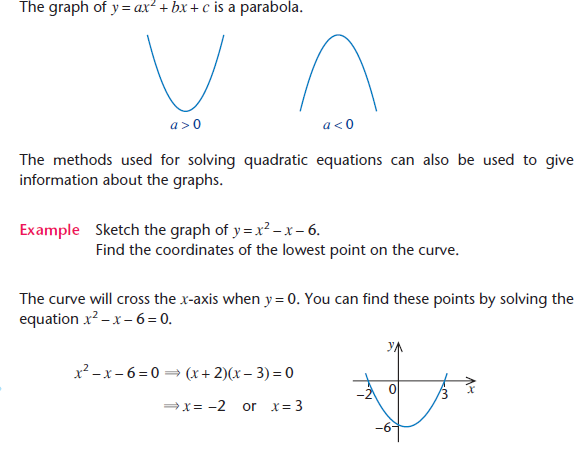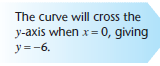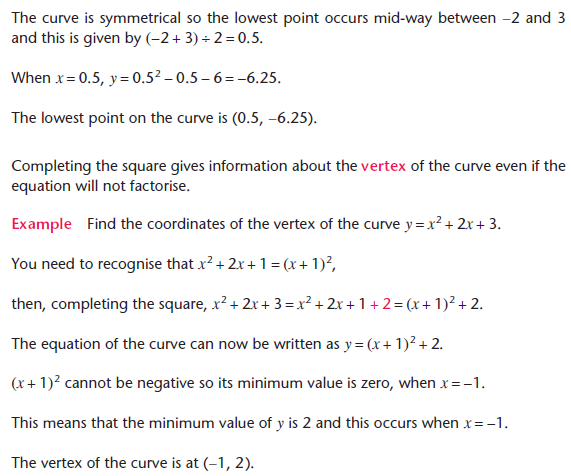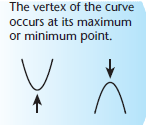This section covers Quadratic Equations.

In algebra, any expression of the form ax2 + bx + c where a ≠ 0 is called a quadratic expression.

It is useful to remember these results of expanding brackets:

(x + a)2 = x2 + 2ax + a2

(x - a)2 = x2 – 2ax + a2

(x + a)(x – a) = x2 – a2

Equations of the form ax2 + bx + c = 0, where a ≠ 0 are quadratic equations.

Some quadratic equations can be solved by factorising the quadratic expression.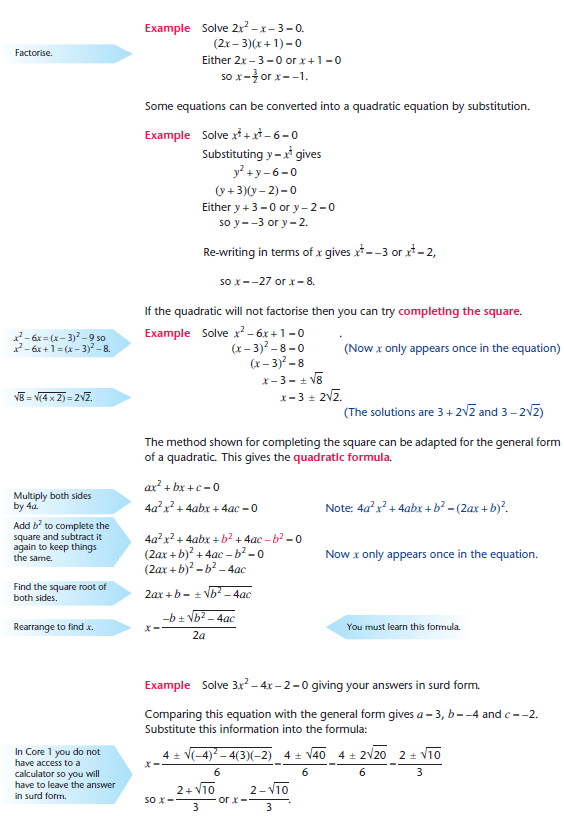Discriminant